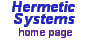Static and Dynamic Allocation
of Multi-Dimensional Arrays in CAn array in C is a region of memory in which the elements (chars, ints, etc.) can be accessed using an index (in a 1-dimensional array, e.g. name) or several indices (in a multi-dimensional array, e.g. names). The first element in a 1-dimensional array x[] is x, in a 2-dimensional array x[][] is x, and so on.

In C you can allocate memory for an array using statements such as:

 ```char name; int nums; double coords char names; double space;```

The index of the last element in a 1-dimensional array is one less than the size of the array (e.g. name if the array is declared using char name). In a multidimensional array the indices of the last element are one less than the sizes of each dimension (e.g. names if the array is declared using char names).

When an array is declared as above, memory is allocated for the elements of the array when the program starts, and this memory remains allocated during the lifetime of the program. This is known as static array allocation.

It may happen that you don't know (at the time of writing the code) how large an array you will need (or how many arrays). In this case it is convenient to allocate an array while the program is running. This is known as dynamic array allocation.

Dynamic allocation of a 1-dimensional array is easily done using the malloc() function. For example, if you wish to allocate an array of 1000 ints then the following code can be used:

 ```#include #include #include void main(void) { int *a; int i=1000; if ( ( a = (int *)malloc(i*sizeof(int)) ) == NULL ) { printf("\nError, memory not allocated.\n"); exit(1); } for ( i=0; i<1000; i++ ) a[i] = i; // ... free(a); } ```

Dynamic allocation of arrays of more than one dimension is not so easily done, because dynamic allocation of an n-dimensional array actually requires dynamic allocation of n 1-dimensional arrays. To allocate a 2-dimensional array you must first allocate memory sufficient to hold all the elements of the array (the data), then allocate memory for pointers to each row of the array. For arrays of more than two dimensions it gets more complicated. To allocate a 3-dimensional array you first allocate memory for the data, then allocate memory for an array of pointers to rows of data within that memory, then allocate memory for an array of pointers to a subset of those pointers. And so on for arrays of higher dimension.